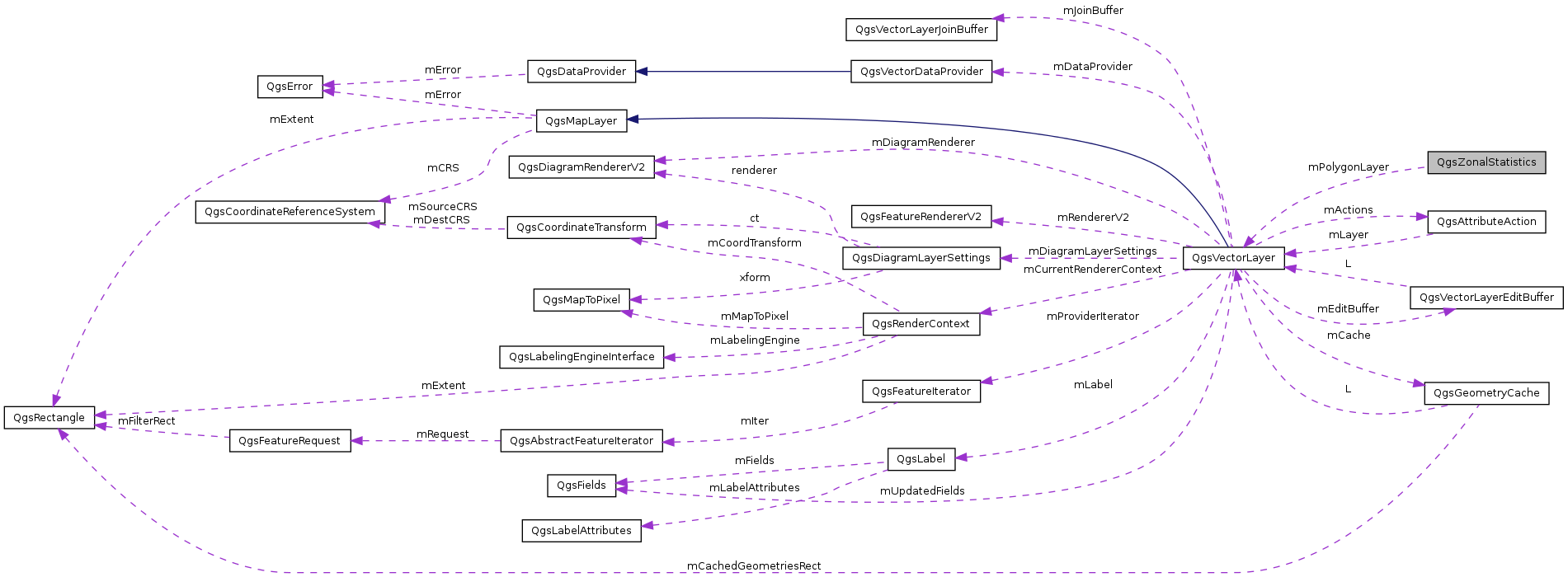QGIS API Documentation  2.0.1-Dufour
QgsZonalStatistics Class Reference

A class that calculates raster statistics (count, sum, mean) for a polygon or multipolygon layer and appends the results as attributes. More...

`#include <qgszonalstatistics.h>`

Collaboration diagram for QgsZonalStatistics:[legend]

## Public Member Functions

QgsZonalStatistics (QgsVectorLayer *polygonLayer, const QString &rasterFile, const QString &attributePrefix="", int rasterBand=1)
~QgsZonalStatistics ()
int calculateStatistics (QProgressDialog *p)
Starts the calculation.

## Private Member Functions

QgsZonalStatistics ()
int cellInfoForBBox (const QgsRectangle &rasterBBox, const QgsRectangle &featureBBox, double cellSizeX, double cellSizeY, int &offsetX, int &offsetY, int &nCellsX, int &nCellsY) const
Analysis what cells need to be considered to cover the bounding box of a feature.
void statisticsFromMiddlePointTest (void *band, QgsGeometry *poly, int pixelOffsetX, int pixelOffsetY, int nCellsX, int nCellsY, double cellSizeX, double cellSizeY, const QgsRectangle &rasterBBox, double &sum, double &count)
Returns statistics by considering the pixels where the center point is within the polygon (fast)
void statisticsFromMiddlePointTest_improved (void *band, QgsGeometry *poly, int pixelOffsetX, int pixelOffsetY, int nCellsX, int nCellsY, double cellSizeX, double cellSizeY, const QgsRectangle &rasterBBox, double &sum, double &count)
void statisticsFromPreciseIntersection (void *band, QgsGeometry *poly, int pixelOffsetX, int pixelOffsetY, int nCellsX, int nCellsY, double cellSizeX, double cellSizeY, const QgsRectangle &rasterBBox, double &sum, double &count)
Returns statistics with precise pixel - polygon intersection test (slow)
QString getUniqueFieldName (QString fieldName)

## Private Attributes

QString mRasterFilePath
int mRasterBand
Raster band to calculate statistics from (defaults to 1)
QgsVectorLayermPolygonLayer
QString mAttributePrefix
float mInputNodataValue
The nodata value of the input layer.

## Detailed Description

A class that calculates raster statistics (count, sum, mean) for a polygon or multipolygon layer and appends the results as attributes.

Definition at line 29 of file qgszonalstatistics.h.

## Constructor & Destructor Documentation

 QgsZonalStatistics::QgsZonalStatistics ( QgsVectorLayer * polygonLayer, const QString & rasterFile, const QString & attributePrefix = `""`, int rasterBand = `1` )

Definition at line 33 of file qgszonalstatistics.cpp.

 QgsZonalStatistics::~QgsZonalStatistics ( )

Definition at line 50 of file qgszonalstatistics.cpp.

 QgsZonalStatistics::QgsZonalStatistics ( )
private

Definition at line 43 of file qgszonalstatistics.cpp.

## Member Function Documentation

 int QgsZonalStatistics::calculateStatistics ( QProgressDialog * p )
 int QgsZonalStatistics::cellInfoForBBox ( const QgsRectangle & rasterBBox, const QgsRectangle & featureBBox, double cellSizeX, double cellSizeY, int & offsetX, int & offsetY, int & nCellsX, int & nCellsY ) const
private

Analysis what cells need to be considered to cover the bounding box of a feature.

Returns
0 in case of success

Definition at line 244 of file qgszonalstatistics.cpp.

Referenced by calculateStatistics().

 QString QgsZonalStatistics::getUniqueFieldName ( QString fieldName )
private

Definition at line 372 of file qgszonalstatistics.cpp.

Referenced by calculateStatistics().

 void QgsZonalStatistics::statisticsFromMiddlePointTest ( void * band, QgsGeometry * poly, int pixelOffsetX, int pixelOffsetY, int nCellsX, int nCellsY, double cellSizeX, double cellSizeY, const QgsRectangle & rasterBBox, double & sum, double & count )
private

Returns statistics by considering the pixels where the center point is within the polygon (fast)

Definition at line 268 of file qgszonalstatistics.cpp.

Referenced by calculateStatistics().

 void QgsZonalStatistics::statisticsFromMiddlePointTest_improved ( void * band, QgsGeometry * poly, int pixelOffsetX, int pixelOffsetY, int nCellsX, int nCellsY, double cellSizeX, double cellSizeY, const QgsRectangle & rasterBBox, double & sum, double & count )
private
 void QgsZonalStatistics::statisticsFromPreciseIntersection ( void * band, QgsGeometry * poly, int pixelOffsetX, int pixelOffsetY, int nCellsX, int nCellsY, double cellSizeX, double cellSizeY, const QgsRectangle & rasterBBox, double & sum, double & count )
private

Returns statistics with precise pixel - polygon intersection test (slow)

Definition at line 328 of file qgszonalstatistics.cpp.

Referenced by calculateStatistics().

## Member Data Documentation

 QString QgsZonalStatistics::mAttributePrefix
private

Definition at line 63 of file qgszonalstatistics.h.

Referenced by calculateStatistics().

 float QgsZonalStatistics::mInputNodataValue
private

The nodata value of the input layer.

Definition at line 65 of file qgszonalstatistics.h.

Referenced by calculateStatistics(), and statisticsFromMiddlePointTest().

 QgsVectorLayer* QgsZonalStatistics::mPolygonLayer
private

Definition at line 62 of file qgszonalstatistics.h.

Referenced by calculateStatistics(), and getUniqueFieldName().

 int QgsZonalStatistics::mRasterBand
private

Raster band to calculate statistics from (defaults to 1)

Definition at line 61 of file qgszonalstatistics.h.

Referenced by calculateStatistics().

 QString QgsZonalStatistics::mRasterFilePath
private

Definition at line 59 of file qgszonalstatistics.h.

Referenced by calculateStatistics().

The documentation for this class was generated from the following files: